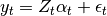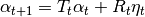# statsmodels.tsa.kalmanf.kalmanfilter.KalmanFilter¶

class statsmodels.tsa.kalmanf.kalmanfilter.KalmanFilter[source]

Kalman Filter code intended for use with the ARMA model.

Notes

The notation for the state-space form follows Durbin and Koopman (2001).

The observation equations isThe state equation isFor the present purposed epsilon_{t} is assumed to always be zero.

Methods

 R(params, r, k, q, p) The coefficient matrix for the state vector in the observation equation. T(params, r, k, p) The coefficient matrix for the state vector in the state equation. Z(r) Returns the Z selector matrix in the observation equation. geterrors(y, k, k_ar, k_ma, k_lags, nobs, ...) Returns just the errors of the Kalman Filter loglike(params, arma_model[, set_sigma2]) The loglikelihood for an ARMA model using the Kalman Filter recursions.